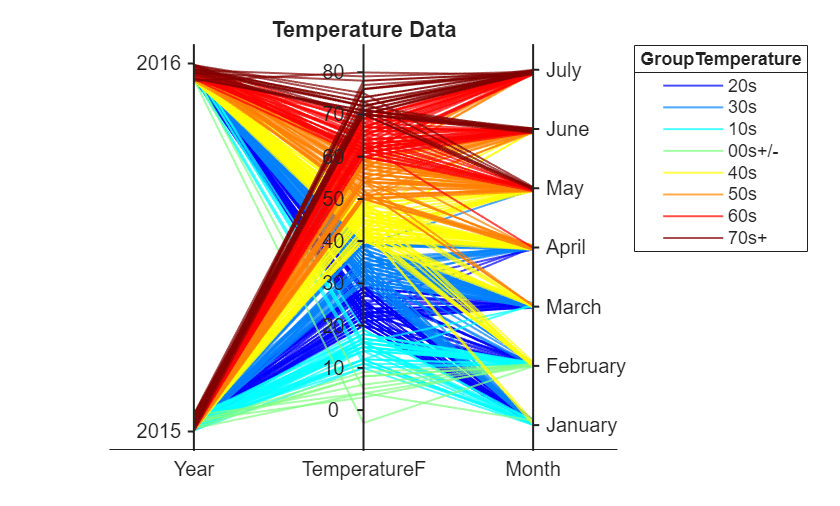Explore Table Data Using Parallel Coordinates Plot

This example shows how to import a file into MATLAB® as a table, create a parallel coordinates plot from the tabular data, and modify the appearance of the plot.

Parallel coordinates plots are useful for visualizing tabular or matrix data with multiple columns. The rows of the input data correspond to lines in the plot, and the columns of the input data correspond to coordinates in the plot. You can group the lines in the plot to better see trends in your data.

Import File as Table

Load the sample file TemperatureData.csv, which contains average daily temperatures from January 2015 through July 2016. Read the file into a table, and display the first few rows.

ans=8×4 table
Year       Month       Day    TemperatureF
____    ___________    ___    ____________

2015    {'January'}     1          23
2015    {'January'}     2          31
2015    {'January'}     3          25
2015    {'January'}     4          39
2015    {'January'}     5          29
2015    {'January'}     6          12
2015    {'January'}     7          10
2015    {'January'}     8           4

Create Basic Parallel Coordinates Plot

Create a parallel coordinates plot from the first few rows of the table. Each line in the plot corresponds to a single row in the table. By default, parallelplot displays all the coordinate variables in the table, in the same order as they appear in the table. The software displays the coordinate variable names below their corresponding coordinate rulers.

The plot shows that the first eight rows of the table provide temperature data for the first eight days in January 2015. For example, the eighth day was the coldest of the eight days, on average.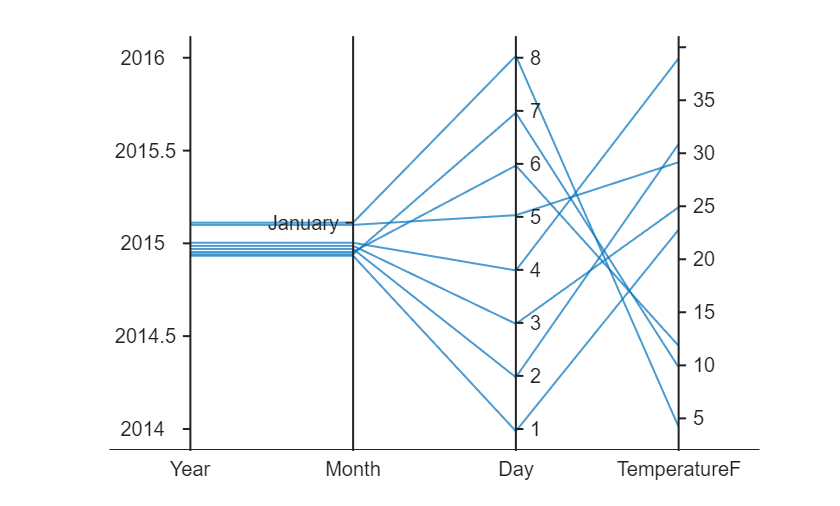To help you interpret the plot, MATLAB randomly jitters plot lines by default so that they are unlikely to overlap perfectly along coordinate rulers. For example, although the first eight observations have the same Year and Month values, the plot lines are not flush with the 2015 tick mark along the Year coordinate ruler or the January tick mark along the Month coordinate ruler. Although jittering affects all coordinate variables, it is often more noticeable along categorical coordinate rulers because it depends on the distance between tick marks. You can control the amount of jittering in the plot by setting the Jitter property.

Notice that some of the tick marks along the Year coordinate ruler are meaningless decimal values. To ensure that tick marks along a coordinate ruler correspond only to meaningful values, convert the variable to a categorical variable by using the categorical function.

tbl.Year = categorical(tbl.Year);

Now create a parallel coordinates plot from the entire table. Assign the ParallelCoordinatesPlot object to the variable p, and use p to modify the plot after you create it. For example, add a title to the plot using the Title property.

p = parallelplot(tbl)
p =
ParallelCoordinatesPlot with properties:

SourceTable: [565x4 table]
CoordinateVariables: {'Year'  'Month'  'Day'  'TemperatureF'}
GroupVariable: ''

Show all properties

p.Title = 'Temperature Data';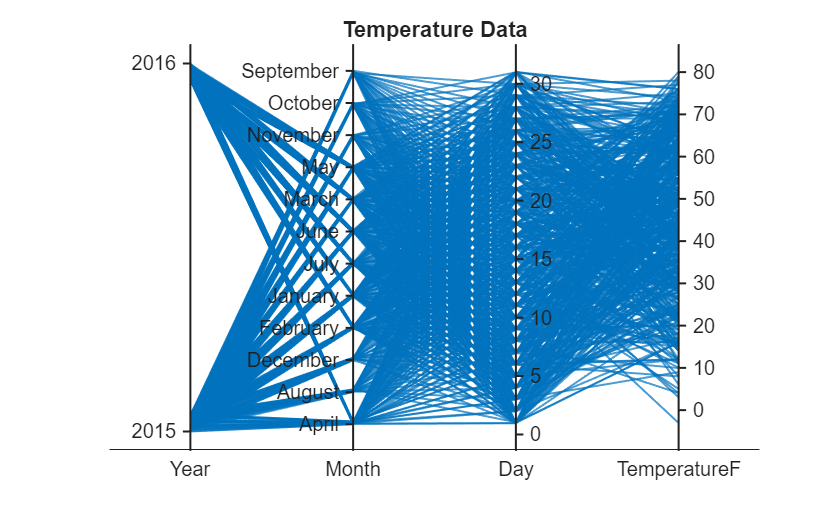Group Plot Lines

Group the lines in the plot according to the Year values by setting the GroupVariable property. By default, MATLAB adds a legend to the plot. You can remove the legend by setting the LegendVisible property to 'off'.

p.GroupVariable = 'Year';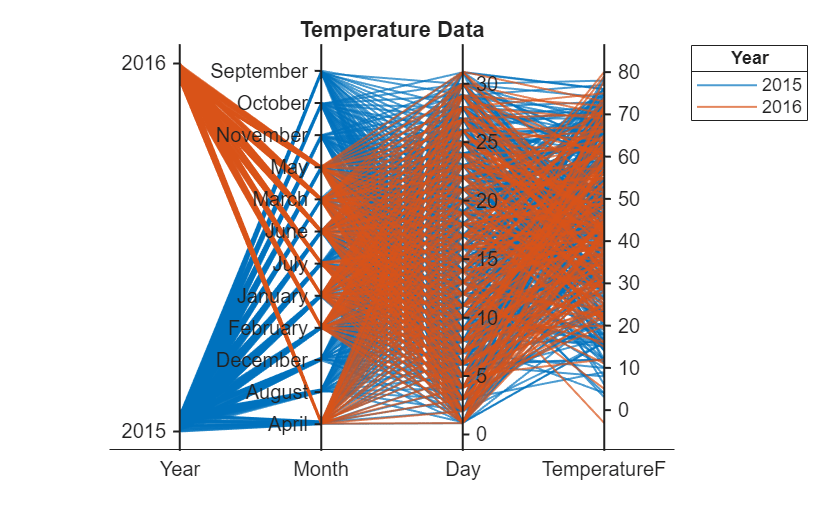Rearrange Coordinate Variables Interactively

Rearrange coordinate variables interactively to compare them more easily and decide which variables to keep in your plot.

Open your plot in a figure window. Click a coordinate tick label and drag the associated coordinate ruler to the location of your choice. The software outlines the selected coordinate ruler in a black rectangle. For example, you can click the Month coordinate tick label and drag the coordinate ruler to the right. You can then easily compare Month and TemperatureF values.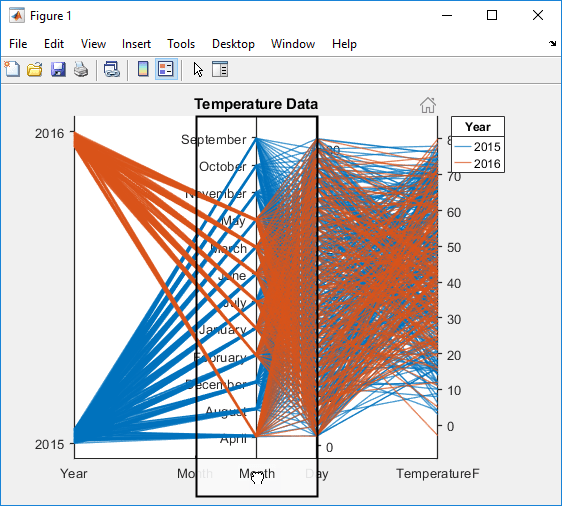When you rearrange coordinate variables interactively, the software updates the associated CoordinateTickLabels, CoordinateVariables, and CoordinateData properties of the plot.

For more interactivity options, see Tips.

Select Subset of Coordinate Variables

Display a subset of the coordinate variables in p.SourceTable and specify their order in the plot by setting the CoordinateVariables property of p.

In particular, remove the Day variable from the plot, and display the TemperatureF variable, which is in the fourth column of the source table, as the second coordinate in the plot.

p.CoordinateVariables = [1 4 2];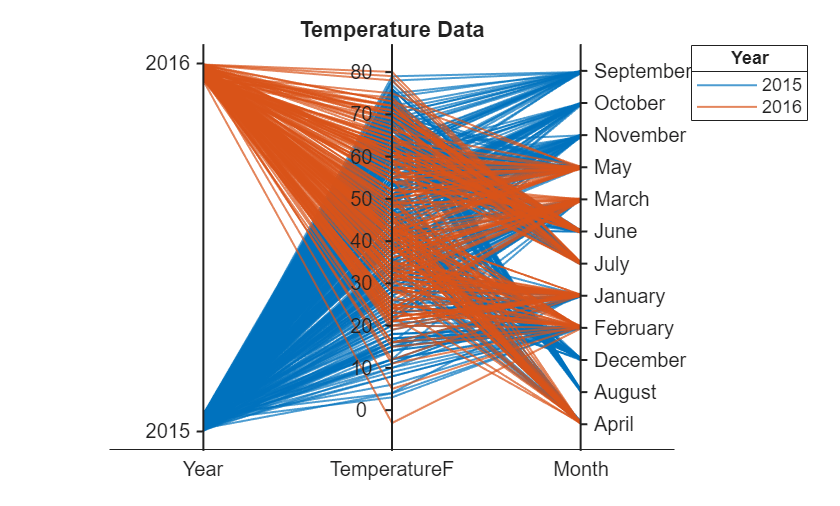Alternatively, you can set the CoordinateVariables property by using a string or cell array of variable names or a logical vector with true elements for the selected variables.

Modify Categories in Coordinate Variable

Display a subset of the categories in Month and change the category order along the coordinate ruler in the plot.

Because some months have data for only one of the two years, remove the rows in the source table corresponding to those unique months. MATLAB updates the plot as soon as you change the source table.

uniqueMonth = {'September','October','November','December','August'};
uniqueMonthIdx = ismember(p.SourceTable.Month,uniqueMonth);
p.SourceTable(uniqueMonthIdx,:) = [];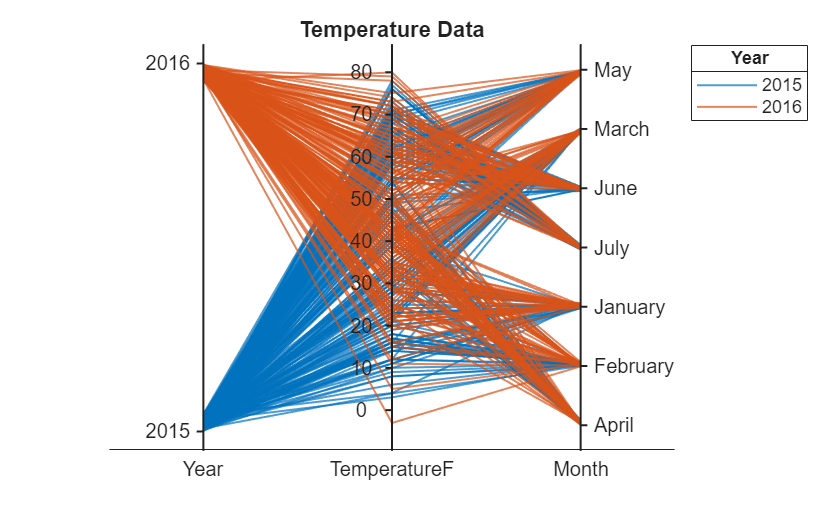Arrange the months in chronological order along the Month coordinate ruler by updating the source table.

categoricalMonth = categorical(p.SourceTable.Month);
newOrder = {'January','February','March','April','May','June','July'};
orderMonth = reordercats(categoricalMonth,newOrder);
p.SourceTable.Month = orderMonth;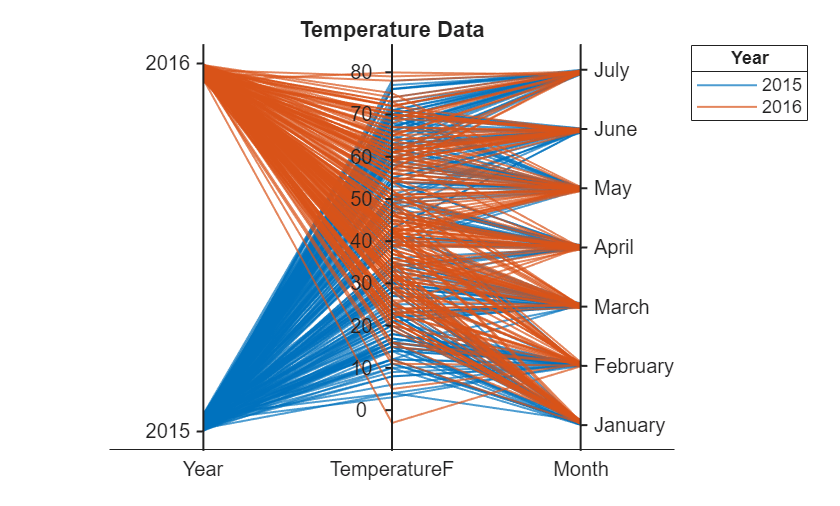Group Plot Lines Using Binned Values

To better visualize the range of temperatures during each month, bin the temperature data by using discretize and group the lines in the plot using the binned values. Check the minimum and maximum temperatures in the source table. Set the bin edges such that they include these values.

min(p.SourceTable.TemperatureF)
ans = -3
max(p.SourceTable.TemperatureF)
ans = 80
binEdges = [-3 10:10:80];
bins = {'00s+/-','10s','20s','30s','40s','50s','60s','70s+'};
groupTemperature = discretize(p.SourceTable.TemperatureF, ...
binEdges,'categorical',bins);

Add the binned temperatures to the source table. Group the lines in the plot according to the binned temperature data.

p.SourceTable.GroupTemperature = groupTemperature;
p.GroupVariable = 'GroupTemperature';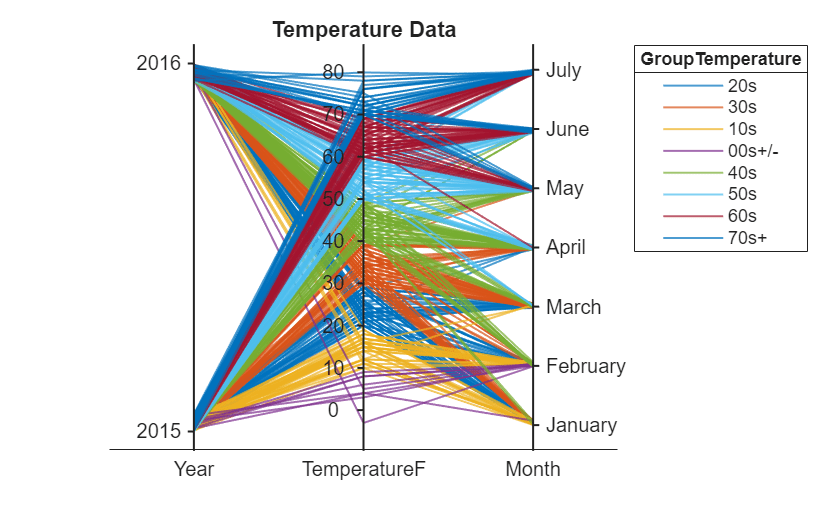Because GroupTemperature includes more than seven categories, some of the groups have the same color in the plot. Assign distinct colors to every group by setting the Color property.

p.Color = jet(8);